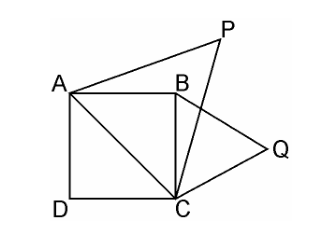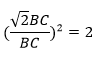Guru

# Prove that the area of an equilateral triangle described on one side of a square is equal to half the area of the equilateral triangle described on one of its diagonals. Q.7

• 0

How i solve the problem of class 10th of chapter Triangles of exercise 6.4. How i solve this question in easy way Prove that the area of an equilateral triangle described on one side of a square is equal to half the area of the equilateral triangle described on one of its diagonals.

Share

1.Given, ABCD is a square whose one diagonal is AC. ΔAPC and ΔBQC are two equilateral triangles described on the diagonals AC and side BC of the square ABCD.

Area(ΔBQC) = ½ Area(ΔAPC)

Since, ΔAPC and ΔBQC are both equilateral triangles, as per given,

∴ ΔAPC ~ ΔBQC [AAA similarity criterion]

∴ area(ΔAPC)/area(ΔBQC) = (AC2/BC2) = AC2/BC2

Since, Diagonal = √2 side = √2 BC = AC⇒ area(ΔAPC) = 2 × area(ΔBQC)

⇒ area(ΔBQC) = 1/2area(ΔAPC)

Hence, proved.

• 0